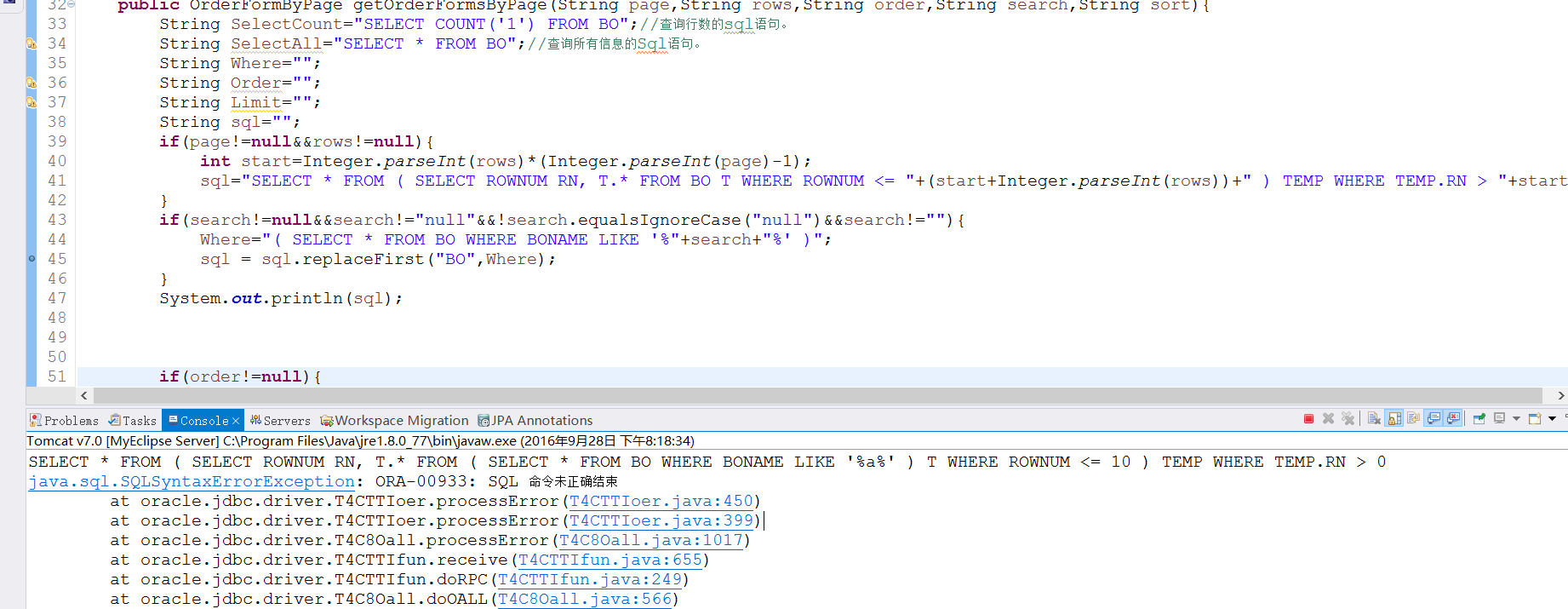ORA-00933: SQL 命令未正确结束问题

`````` if(page!=null&&rows!=null){
int start=Integer.parseInt(rows)*(Integer.parseInt(page)-1);
sql="SELECT * FROM ( SELECT ROWNUM RN, T.* FROM BO T WHERE ROWNUM <= "+(start+Integer.parseInt(rows))+" ) TEMP WHERE TEMP.RN > "+start;
}
if(search!=null&&search!="null"&&!search.equalsIgnoreCase("null")&&search!=""){
Where="( SELECT * FROM BO WHERE BONAME LIKE '%"+search+"%' )";
sql = sql.replaceFirst("BO",Where);
}
``````

myeclipse 执行结果：
SELECT * FROM ( SELECT ROWNUM RN, T.* FROM ( SELECT * FROM BO WHERE BONAME LIKE '%a%' ) T WHERE ROWNUM <= 10 ) TEMP WHERE TEMP.RN > 0

java.sql.SQLSyntaxErrorException: ORA-00933: SQL 命令未正确结束1个回答Derek_yule 不行。但是SELECT * FROM ( SELECT ROWNUM RN, T.* FROM BO T WHERE ROWNUM <= 10 ) TEMP WHERE TEMP.RN > 0原来是都是可以执行的。但是更改了Where之后就不能用了。但是把改过where的语句放在SQL Developer运行一点事都没有。